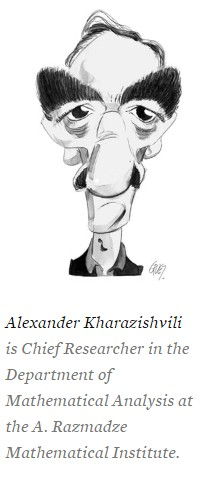# Cantor’s Diagonalization Method

Alexander Kharazishvili in Inference Review:Among various philosophical categories, the finite and the infinite are of pivotal importance. The relationship between them is so close, sophisticated, and profound that neither can be perceived and studied without appealing to the other. Both notions are the subject of extensive mathematical research. Modern set theory is entirely devoted to the study of the most general properties of finite and infinite families of objects.

A set of objects is finite if there exists a one-to-one correspondence, or bijection, between the set and some natural number n. It is assumed that nis identified with the set of all strictly smaller natural numbers. It follows that 0 is identical to the empty set , and 1 coincides with the one-element set {}, while 2 is equal to {,{}}, and so on. If a set of objects is not finite, it is infinite. For example, it is a straightforward to demonstrate that the set N of all natural numbers is infinite.

Galileo offered some useful remarks about the paradoxical nature of infinite sets in the seventeenth century.1 There is a one-to-one correspondence between the natural numbers and their squares. N is equinumerous with a proper part of itself. This is impossible for a finite set of objects.

Bernard Bolzano suggested defining infinite sets as those sets that are equinumerous with their proper subsets.2 This is the definition adopted by Richard Dedekind.3 All sets satisfying this definition are now known as infinite in the Dedekind sense: D-infinite sets.4

More here.